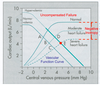# Cardiovascular lecture 2: interaction btwn the heart and circulation week 3 Flashcards Preview

## CV M1 > Cardiovascular lecture 2: interaction btwn the heart and circulation week 3 > Flashcards

Flashcards in Cardiovascular lecture 2: interaction btwn the heart and circulation week 3 Deck (15):
1

## What veins are considered to be in the central venous compartment? What is venous return?

### central veins compartment: great veins in the thorax and in the right atriumvenous return: flow (ml/min) returning to the CVP from teh peripheral venous compartment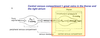2

3

4

## What determines filling of the ventricles?

### Filling of the RV is dtermined by the pressure present in the RA which is in turn determined by CVP. The RV, pulmonary circulation, and LA constitute an essentailly passive conduit conveying blood to the LV (passivein the sense that it does not participate in any of the reflex responses that affect flow in the systemic circulation). if pressure is peripheral venous compartment is 7 mmHg, CVP must be lower when CVP is high: increased filling of RV: increased fiber stretch increased filling of the RV leads to increased filling of LV bc connected. which means increased preload and increased output on both the left and right.It is common, in talking about cardiac function, to imply that the left ventricular cardiac output is determined by central venous pressure since any change that occurs in the right atrium is passed along passively to the left atrium and changes left ventricular filling. We will, therefore, always consider ventricular filling on the left side to be determined by CVP and atrial pressure on the right side even though technically it’s the left atrial pressure that directly determines this.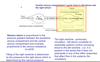5

6

7

8

## We already know 2 points on the vascular function curve: when CO is 5 L/min, CVP is 2 mmHg. When CO is 0, CVP is 7 mmHg. Slowing the heart does the same thing as stopping it (increases CVP), just not to as great an extent. The vascular function curve is simply the line which reflects the fact that when you slow or accelerate the heart, the CVP rises or falls, respectively.Consider our arrested heart. Restart the heart but make CO equal to 1 L/min. What happens to the venous and arterial flows and pressures?When does the pressure difference btwn the arterial and venous side cease to rise (reaches "set" points)?

### At the instant the heart begins to beat the pressure gradient from the arterial side to the venous side across the system is 0 and no blood is flowing across the system at this point. However, blood is being removed from the veins through the heart.Hence, as the heart restarts, the pressure in the veins (CVP) falls. At the same time, the pressure in the arteries (MAP) rises and the increment in MAP is 19 times greater than the decrement in CVP as the same volume of blood is transferred between the systems (again, because of the difference compliance between the two comprtments). The pressure difference (ΔP) will continue to rise until the flow through the system is equal to the CO: ΔP=Q(R) ΔP=1 L/min(20 mmHg/L/min) ΔP =20 mmHg This gradient can be achieved through a 19 mmHg rise in MAP (to 26 mmHg) and a 1 mmHg fall in CVP (to 6 mmHg). Hence at a CO of 1 L/min we have a CVP of 6 mmHg on our vascular function curve.The preceeding calculation can be done for any given CO. The result will be a line which is defined as the vascular fxn curve (see pg 176 of notes). Note that this calculation assumes there are no reflexes. This concept demonstrates only a part of circulatory control.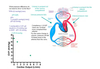9

## What happens to the vascular function curve for hemorrhage and transfusion?

### The vascular fxn curve is profoundly affected by blood volume. The mean circulatory pressure is normally 7 mmHg (i.e. when the heart is stopped, both the arterial and venous side come to 7mmHg). If you decrease the total volume of blood at this point, the pressure will obviously decrease (Figure 2.8 at CO=0). Similarly, volume expansion would mean an increase. Consider the case of volume expansion such that the mean circulatory pressure becomes 9 mmHg e.g. “Transfusion” in Figure 2.8, top). Start with the heart stopped. A sudden increase in CO from 0 to 1 L/min at a resistance of 20 mmHg/L/min means that ΔP will have to increase to 20 mmHg (calculated exactly as above with the control curve using the new mean circulatory pressure – try it). Once again, the proper gradient is achieved by increasing the MAP 19 mmHg (this time to 9 mmHg +19 mmHg or 28 mmHg total) and a decrease of 1 mmHg (this time to 9 mmHg – 1 mmHg or 8 mmHg, Figure 2.8, top). You can see, therefore, that the vascular function curves for volume expansion and for hemorrhage are simply parallel lines which are shifted upward or downward, respectively. Hemorrhage is simply the same calculation starting at a lower mean circulatory pressure.10

## What changes to the vascular function curve are observed when TPR is increased (through vasoconstriction of arterioles)?

### Note that the arterioles contain only a minimal amount of blood volume (about 3%).Therefore the mean circulatory pressure (CVP at CO=0) will not change with arteriolar constriction or dilation. I can stop the heart and give a patient a vasodilator or a vasoconstrictor and, assuming it has most of its effect at the arterioles, it will have no significant effect upon the pressure (7 mmHg).Now let’s once again, restart our arrested heart to a CO of 1 L/min but do it with an increased resistance to 40 mmHg/L/min. Now the ΔP can be calculated: ΔP=Q(R) ΔP=1 (40 mmHg/L/min) ΔP=40 mmHg When our resistance was 20, the ΔP was 20 mmHg but now it is twice as great. To achieve this change in pressure, twice the volume must be transferred from the venous side to the arterial side and the decrease in venous pressure is twice as great (to 5 mmHg instead of 7 mmHg). Hence, vasoconstriction and vasodilation change the slope of the vascular function curve without changing the mean circulatory pressure. Note in the figure that at the same pressure, a lower CO is achieved through vasoconstriction as compared to vasodilation. See pg 180 of course notes.11

## What is the cardiac function curve? What intrinsic property of the heart is it telling of?

### As mentioned previously in accordance with Starling’s Law, CO is intimately associated with CVP. Higher CVP results in greater ventricular filling and stretch of the ventricular muscle fibers causing increased SV. This relationship between CO and CVP is known as the cardiac function curve12

## So, which curve is right and operates in the body?### So how can CVP go down with as CO goes up in Figure 2.9 and up as CO goes up in Figure 2.10? The difference may be illustrated in Figure 2.11. It is a diagram of an isolated heart preparation in which it is possible to vary: (1) ventricular filling pressure (CVP or, equivalently, RAP) and (2) the cardiac output (CO) by varying the heart rate with a pacemaker. The root of the difference between these two curves lies between these two variables. If one varies the CVP and measures the CO, the cardiac function curve results. If, on the other hand, one varies the CO and measures the CVP, the vascular function curve results. The key to understanding the physiological significance of these curves is to understand that in the body, both variables change, not just one or the other.13

## Consider the attached picture. Points A and B are equilibrium points. How are they achieved?### These two relationships, the vascular and cardiac function curves, define an extremely simple physiological feedback loop. For instance, let’s starts at a normal CO of 5 L/min and a CVP of about 2 mmHg. To make it simple, we’ll say that physiological reflexes such as the ones described in the next lecture are not intact. Now let’s increase the blood volume through a transfusion. As a result, CVP suddenly increases (1 on Figure 2.12). Immediately upon the rise in CVP, the CO rises, say to 7.5 L/min (effect of the cardiac function curve, 2 on Figure 2.12). The rise in CO results in a gradual decrease in CVP (vascular function curve, 3 on Figure 2.12). The resulting decrease in CVP, on the other hand, gradually decreases the CO. Eventually a new steady state is established where the CO is somewhere greater than the 5 L/min we started with but somewhere not as high as the 7.5 L/min it was originally increased to upon blood transfusion. This operating point (or equilibrium point) defines where the steady state CO and the CVP will rest under a given condition is. It is defined by the intersection between the vascular function curve (Figure 2.7 turned on its side) and the cardiac function curve (Figure 2.10).14

## Consider the attached figure. Explain (separately) what occurs to reach equlibrium points C and D.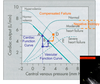### Acute heart failure may be described in its simplest  form as a decrease in CO at any given filling pressure (CVP) or a shift downward in the cardiac function curve (1 on Figure 2.13). You will recall that this essentially is the definition of negative inotropy because the filling pressure determines cardiac stretch (i.e. cardiac fiber length). In acute heart failure the resulting decrease in CO causes an increase in CVP (Vascular function curve, 2 on Figure 2.13). This leads to an increase in CO (cardiac function curve, 3 on Figure 2.13) which leads to a decrease in CVP (4 on Figure 2.13) and so on until we reach our new equilibrium point. The end result is a shift in the equilibrium point in the figure from point A to point B where the CVP is increased (possibly resulting in edema) because of the depressed CO.  If the case in Figure 2.13 (top) becomes chronic, the body will increase the blood volume through fluid retention by the kidneys. This results in a parallel upward shift in the vascular function curve (essentially the same as the transfusion). The equilibrium point moves to yet another equilibrium point (D on Figure 2.13, bottom) at a higher CO, thus largely compensating for the decreased cardiac function by raising the cardiac output to nearly normal. This is known as compensated heart failure.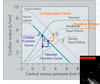15

## Consider the attached figure. What is this new equilbrium point a result of? What are the effects as it relates to MAP?